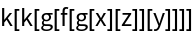#Function Repository Resource:

# SKCombinatorCompile

Represent an expression in terms of the S and K combinators

Contributed by: Wolfram Research
 ResourceFunction["SKCombinatorCompile"][expr,vars] give a representation of expr with respect to vars in terms of the S and K combinators.

## Details and Options

The second argument to ResourceFunction["SKCombinatorCompile"] can be a single variable or list of variables.
ResourceFunction["SKCombinatorCompile"] can take the following option:
 "SKGlyphs" {CombinatorS,CombinatorK} symbols used to specify combinators

## Examples

### Basic Examples (2)

Represent an expression in terms of the S and K combinators:

 In:=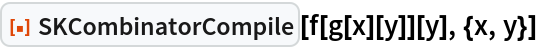Out=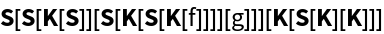Recover the original expression by applying the combinator to variables and transforming:

 In:=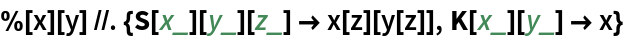Out=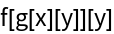### Scope (3)

Convert an expression with one variable:

 In:=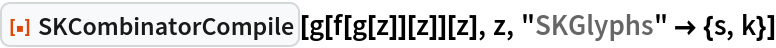Out=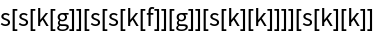Convert an expression with multiple variables:

 In:=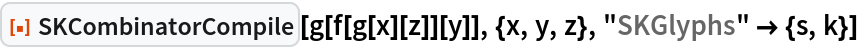Out=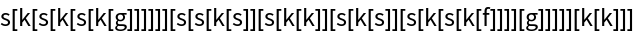Convert an expression where the variables specified are absent in the expression:

 In:=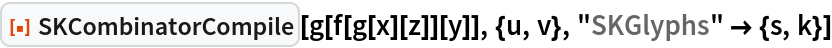Out=